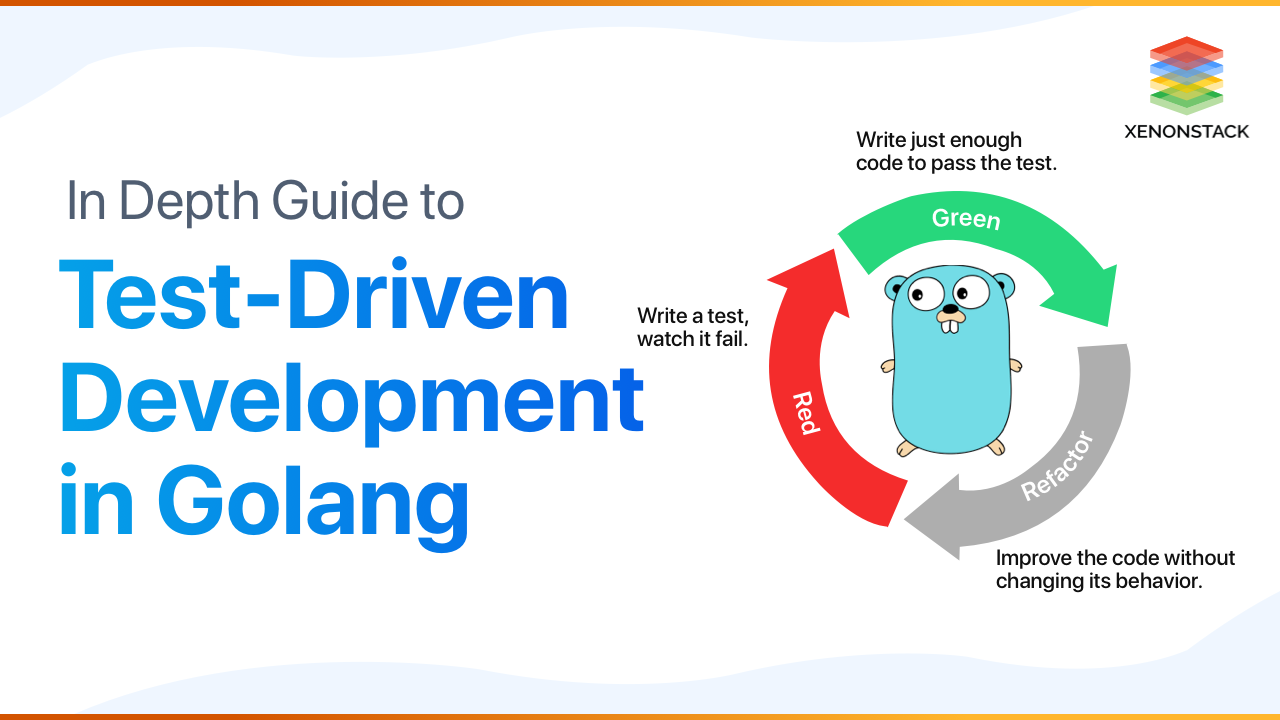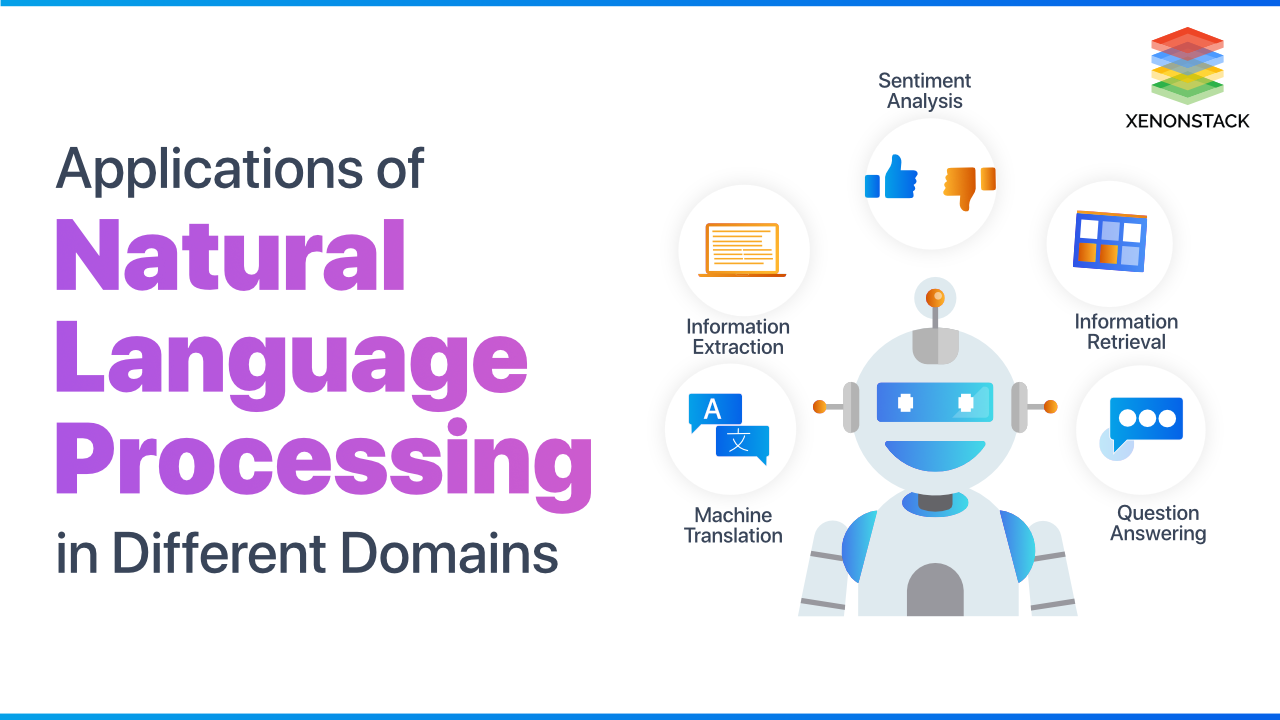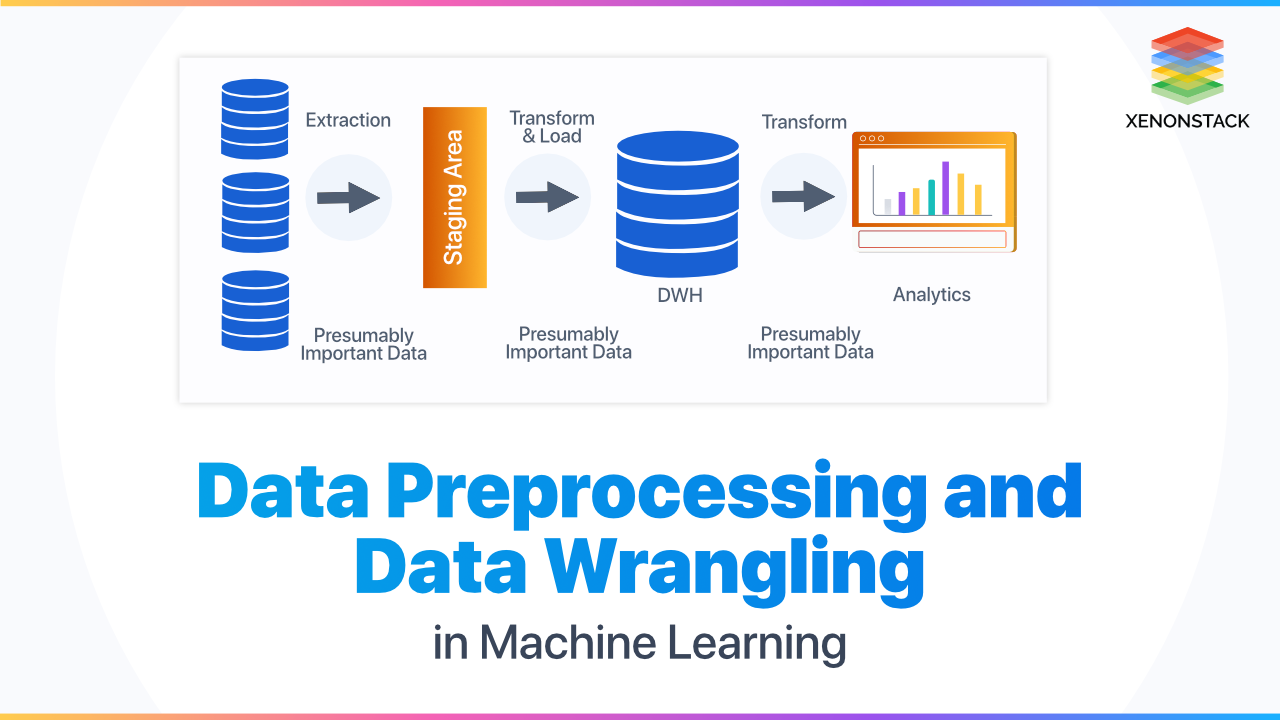#### XenonStack Recommends

SubscriptionThanks for submitting the form.## What is a TensorFlow, anyway?

If you are a fan of Machine Learning/Data Science/ Artificial Intelligence, you cannot miss TensorFlow. The name defines itself. Tensor it is nothing, but a multidimensional array and open-source library for computation. In today's world, Google's TensorFlow is the most popular deep learning library in the world. The tools or search engines that work on translation, image captioning, recommendation, prediction work on TensorFlow. TensorFlow Architecture is used at the Huge dataset to provide the best experience to the Users. TensorFlow applications can train and run the neural networks for recurrent neural networks, written digit classification, image recognition, NLP (Natural Language Processing), and PDE (Partial Differential Equation) based simulations.

## TensorFlow in Neural Network

A neural network is a ' Set of Functions' or a ' Group of Algorithms' that determines the output based on previous learning or prior knowledge. For Example, if an Artificial Neural Network is developed to recognize handwritten alphabets, then we must provide a set of handwritten alphabets that will act as prior knowledge for the machine. So, the problem is to recognize the handwritten image from a dataset of images. We have a subset of the images for training and the remaining images to test the neural model and to do so first. We download the train and test files. The dataset comprises a zipped file of all images in the dataset and both train.csv and test.csv as well have the name of the corresponding train and test images. To implement the same task, we must follow the code given below:

### Step 1: First, we import all the necessary libraries

```%pylab inline

import os

import tensorflow as tf

import numpy as np

import pandas as pd

from scipy.misc import imread

from sklearn.metrics import accuracy_score
```

### Step 2: Then we set a seed value to remove randomness

```# To stop potential randomness

seed = 100

ran = np.random.RandomState(seed)
```

### Step 3: Select the CSV file directory to import the dataset

```root = os.path.abspath('../..')

data = os.path.join(root, 'data')

sub = os.path.join(root, 'sub')

#checking for existance

os.path.exists(root)

os.path.exists(data)

os.path.exists(sub)```

### Step 4: Read the CSV file along with the appropriate labels

```traindata = pd.read_csv(os.path.join(data, 'Train', 'train.csv'))

submission = pd.read_csv(os.path.join(data_dir, 'Submission.csv'))

### Step 5: Check the format of data

```img_name = ran.choice(train.filename)

filepath = os.path.join(data_dir, 'Train', 'Images', 'train', img_name)

img = imread(filepath, flatten=True)

pylab.imshow(img, cmap='gray')

pylab.axis('off')

pylab.show()```

### Step 6: Store All the images as numpy Array

```temp = []

for img_name in train.filename:

image_path = os.path.join(data, 'Train', 'Images', 'train', img_name)```
```    img = imread(image_path, flatten=True)

img = img.astype('float32')

temp.append(img)

train_x = np.stack(temp)

temp = []

for img_name in test.filename:

image_path = os.path.join(data, 'Train', 'Images', 'test', img_name)

img = imread(image_path, flatten=True)

img = img.astype('float32')

temp.append(img)

test_x = np.stack(temp)```

### Step 7: Train and Test Machine Learning Model

As we need to train and test the machine learning model so we will split the data set In training and testing sets: - here we divided the dataset in 70:30 ratio
```split_size = int(traindata_x.shape*0.7)

traindata_x, val_x = traindata_x[:split_size], traindata_x[split_size:]

traindata_y, val_y = traindata.label.values[:split_size], traindata.label.values[split_size:]```

### Step 8: Convert Class Labels

These functions are used to convert class labels from scalars to one hot-vector, To convert values to range from 0 to 1.
```def dense_to_one_hot(labels_dense, num_classes=10):

num_labels = labels_dense.shape

index_offset = np.arange(num_labels) * num_classes

labels_one_hot = np.zeros((num_labels, num_classes))

labels_one_hot.flat[index_offset + labels_dense.ravel()] = 1

return labels_one_hot

def preproc(unclean_batch_x):

temp_batch = unclean_batch_x / unclean_batch_x.max()

return temp_batch```

### Step 9: Batch and Return Appropriate Format

This function will create a batch with random samples and return the appropriate format.
```def batch_creator(batch_size, dataset_length, dataset_name):

batch_mask = ran.choice(dataset_length, batch_size)

batch_x = eval(dataset_name + '_x')[[batch_mask]].reshape(-1, input_num_units)

batch_x = preproc(batch_x)

if dataset_name == 'train':

batch_y = eval(dataset_name).ix[batch_mask, 'label'].values

batch_y = dense_to_one_hot(batch_y)

return batch_x, batch_y
```

```input_units = 28*28                      # number of neurons in each layer

hidden_units = 500

output_units = 10

x = tf.placeholder(tf.float32, [None, input_units])     # define placeholders

y = tf.placeholder(tf.float32, [None, output_units])```
```epochs = 5                              # set remaining variables

batch_size = 100

learning_rate = 0.01

weights = {

'hidden': tf.Variable(tf.random_normal([input_units, hidden_units], seed=seed)),

'output': tf.Variable(tf.random_normal([hidden_units, output_units], seed=seed)) }

biases = {

'hidden': tf.Variable(tf.random_normal([hidden_units], seed=seed)),

'output': tf.Variable(tf.random_normal([output_units], seed=seed))

}```

### Step 10: Define Neural Network

Now We define a neural network that contains three layers (input, hidden, and the output). The number of neurons in the input and output is fixed, as the input is our 28 x 28 image and output is a 10 x 1 vector representing the class. Now we create the computational graph of the neural networks and define the cost of the neural network. We use a Gradient Descent algorithm as the optimizer of TensorFlow and initialize the variables.
```hidden_layer = tf.add(tf.matmul(x, weights['hidden']), biases['hidden'])

hidden_layer = tf.nn.relu(hidden_layer)

output_layer = tf.matmul(hidden_layer, weights['output']) + biases['output']

cost = tf.reduce_mean(tf.nn.softmax_cross_entropy_with_logits(output_layer, y))

init = tf.initialize_all_variables()```

### Step 11: Run the neural network by creating a session

```with tf.Session() as ses:

ses.run(init)

for epoch in range(epochs):

avg_cost = 0

total_batch = int(traindata.shape/batch_size)

for i in range(total_batch):

batch_x, batch_y = batch_creator(batch_size, traindata_x.shape, 'train')

_, c = ses.run([optimizer, cost], feed_dict = {x: batch_x, y: batch_y})```

### Step 12: To test the model

`img_name = rng.choice(test.filename)`
```filepath = os.path.join(data_dir, 'Train', 'Images', 'test', img_name)

img = imread(filepath, flatten=True)

test_index = int(img_name.split('.')) - 49000

print "Prediction is: ", pred[test_index]

pylab.imshow(img, cmap='gray')

pylab.axis('off')

pylab.show()```

## Why TensorFlow Architecture is in Demand?

There's a heavily popular thing among the machine learning developers; they are crowding toward a tool called TensorFlow, which promotes some of the vital work essential in developing and managing training data sets in ML. Including some of the big brands that are switching to TensorFlow Architecture for machine learning, the demand is visible. The question is, why TensorFlow? TensorFlow is the best library as it is built to be convenient for everyone. TensorFlow library combines various APIs to create a deep learning architecture like CNN (Convolutional Neural Network) or RNN (Recurrent Neural Networks). TensorFlow Architecture is based on graph computation. it acknowledges the developer to imagine the structure of the neural network with Tensorboard. DevOps with TensorFlow is helpful to detect the errors.

## TensorFlow Architecture and Applications

To grasp the concept of architecture, we will discuss the major components of TensorFlow.

The Loaders are the continuation point for adding algorithms and data backends. TensorFlow is one such algorithm backend that can be used for Machine Learning Models deployment with Tensorflow.

### Servables

TensorFlow Servables are the instances that clients apply to perform the computation. A single servable might add anything from a lookup table to a single model to a tuple of deducing models.

### Servable Versions and Streams

By using TensorFlow serving, we can handle multiple versions of servables. At serving time, clients may inquire for either the newest version or a version id, for a specific model. The Stream is managed by using tensor APIs which are used for distributed runtime.

### Models

A TensorFlow serving describes a model as one or a model as multiple servables. A servable can serve as a section of a model.

### Batcher

Batcher significantly decreases the cost of implementing inference, mainly in the presence of hardware accelerators such as GPU. The important uses of the library include classification, perception, understanding, discovering, prediction, and creation. These elements help developers to execute tasks such as Voice recognition, text recognition, Video detection, and many others as well.

## Why do We Use TensorFlow With Python?

In generating applications, developers can use either C++ or Python for TensorFlow. Python is probably the most convenient language for a large scale of data science and machine learning developers, and it is also easy to combine and hold control over the C++ backend. With the primary model of TensorFlow with Python, the execution of Python is not that great. It is also a plus that NumPy offers it ease to perform pre-processing in Python even with a high performance before serving it into TensorFlow for the CPU-heavy things.
Manufacturing Data Analytics Platform is necessary to compete in the manufacturing industry. Click to explore about, Building ML Analytics Platform

## Why is TensorFlow Better Than Any Other Framework?

TensorFlow Architecture competes with some of the well-known frameworks like PyTorch, CNTK, and MXNet, and many others which are known to execute the same task. TensorFlow Architecture works on a static graph computational approach, which is a programmatic way to create networks. According to the developers, the programmatic structure is like 'for loop' are used to develop deeper networks or develop Recurrent Neural Networks (RNN) in fewer lines of code. This shows that developers who know to code or prefer a programmatic path for developing neural networks libraries like TensorFlow.

## What are the advantages of TensorFlow?

• TensorBoard: TensorBoard is an interface which is used to visualize the data, graph, and tools for error detection.
• Graphs: TensorFlow architecture follows define then run, which refers to the static graph computation approach. TensorFlow has good computational graph visualization, which is better than other libraries.
• Enterprise Centric: TensorFlow is a High-performance Framework which is better than other frameworks available in the market.  TensorFlow has a Unique approach that allows observing the training process of the neural models and tracking various metrics. TensorFlow is the framework that has excellent community support.
• Customer-Centric: TensorFlow architecture provides you TensorBoard, TensorFlow lets you perform subparts of a graph which provides it with an upper hand as you can begin and recover discrete data onto an edge and detect the errors using TensorBoard. TensorFlow is extremely parallel and created to use different backend Software TensorFlow contains data and model parallelism so that you can divide the model into segments and run them parallelly. TensorFlow applications have a more electric compile time than Some of the other frameworks like theano etc.
• Supply Centric: The libraries can be deployed on a range of hardware machines, originating from cellular tools to computers with involved setups.
The unique method enables monitoring the training development of neural models and tracking specific metrics.

## What are the challenge of TensorFlow?

• TensorFlow's compile time is better than other frameworks, but it runs dramatically more inactive than other frameworks.
• TensorFlow applications do not support any other GPU, and Only Nvidia GPU is supported.
• To work with TensorFlow, it needs elementary knowledge of high-level calculus and linear algebra along with a stable understanding of machine learning.
• Some machine learning frameworks support more kinds of models.
• Sometimes it is a Drawback that the only fully supported coding language is Python.Our solutions cater to diverse industries with a focus on serving ever-changing marketing needs. Click here for our Machine Learning Services

## How to Optimize TensorFlow Models?

In neural networks, model size matters. Smaller models utilize shorter memory, less storage, and network bandwidth, and they load quicker. In some situations, hardware memory restriction or service limitations may impose a limit on model size. Here are several ways to optimize the model in a better way:
• Pruning: Remove unused nodes in the forecast track and the products of the graph, mixing duplicate nodes.
• Folding: Detect any sub-graphs in the neural model that regularly estimate constant expressions and swap them with those constants.
• Quantization: Optimizations are driven by decreasing the precision of the parameters. The compression is done from their training-time 32-bit floating-point illustrations to much less and efficient 8-bit integer representations.
• Freezing: Turn the parameters stored into a checkpoint repository of the stored model into constants stored straight in the model graph. This decreases the overall size of the model.

## Conclusion

TensorFlow software is so widespread that, today, it is impossible to understand the development of artificial intelligence without its contribution. It is an open-source library developed by Google whose purpose is to extend the use of deep learning to a very wide range of tasks. TensorFlow is written in C ++ and Python, AND with APIs available to R users!

Thanks for submitting the form.## Related blogs and ArticlesData Science

### Golang Unit Testing and Testing Best PracticesData Science

### Applications of Natural Language Processing For BusinessesData Science

### Data Preprocessing and Data Wrangling in Machine Learning

Thanks for submitting the form.